This section is Section 2 of 3.

Speed as well as accuracy is important in this section. Work quickly, or you might not finish the paper. There are no  penalties for incorrect responses, only marks for correct answers, so you should attempt all 27 questions. Each question is worth one mark.

You must complete the answers within the time limit. Calculators are NOT permitted.

Good Luck!

Note – if press “End Exam” you can access full worked solutions for all past paper questions

The diagram shows a section through part of a healthy human.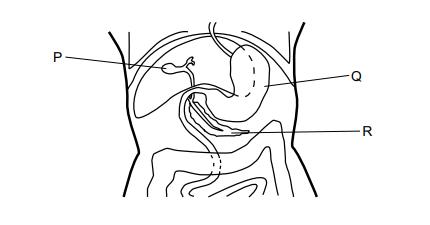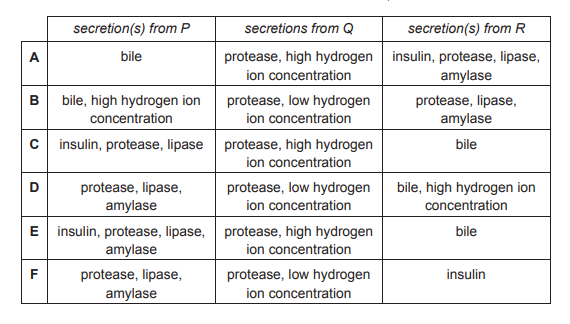1. Which row in the table shows the correct secretions from P, Q and R?
1
0

## Explanation

P is the gallbladder, Q is the stomach and R is the pancreas. The gallbladder stores bile, the stomach secretes protease and HCl and the pancreas secretes insulin, protease, lipase and amylase. So A is the answer.

Post Comment

The diagram shows an electrolysis experiment.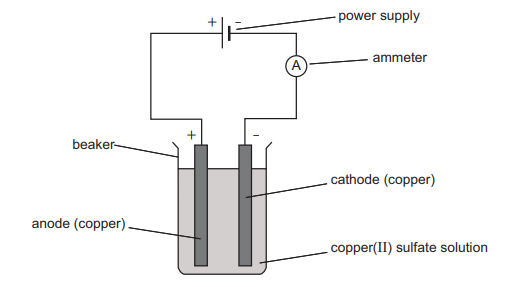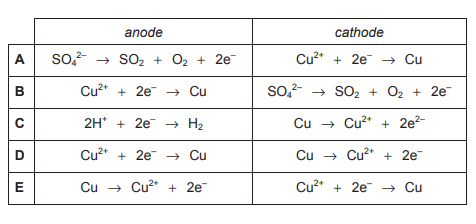2. Which reaction occurs at the anode and which reaction occurs at the cathode for this experiment?
2
0

## Explanation

Oxidation occurs at the anode and reduction at the cathode. Copper has a lower reactivity than both hydrogen and sulfate ions, so copper will be oxidised (lose electrons) at the anode and reduced (gain electrons) at the cathode.

Post Comment

Below are four statements about electromagnetic waves.

1 Microwaves have a smaller wavelength than visible light.

2 The speed of visible light in a vacuum is higher than the speed of other types of electromagnetic wave.

3 Gamma rays have the largest wavelength of any electromagnetic wave.

4 Radio waves are used in hospital radiography to look for broken bones

3. Which of the statements are correct?
0
0

## Explanation

Microwaves have the second largest wavelength (after radiowaves), so statement 1 is incorrect. All EM waves travel at 300,000,000 metres per second (m/s) through a vacuum, so 2 is incorrect. As stated before, radiowaves have the largest wavelength, so 3 is incorrect. Since there is no option for statement 4 to be correct on its own, all 4 statements must be incorrect.

Post Comment

Which one of the following is equivalent to (√5-2)² ?

4.
0
0

## Explanation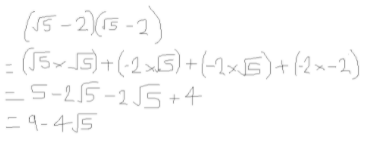Post Comment

SCID is an inherited condition in humans. People with some types of SCID are unable to make a functional enzyme necessary for the production of healthy white blood cells.

A scientist studying these types of SCID compared features of the DNA and the structure of the enzyme in people who have this condition and people without SCID.

1 the allele of the gene coding for the enzyme

2 the order of amino acids in the enzyme

3 the order of bases in the gene coding for the enzyme

4 the shape of the active site of the enzyme

5. Which of the following features may have been different?
0
0

## Explanation

If the allele of the gene coding for the enzyme was different, the function of the enzyme may also be different, so 1 is correct. The different allele could change the amino acid sequence in the enzyme, so 2 is also correct. The order of bases in the gene coding for the enzyme will determine the amino acid sequence, so 3 is correct. A change in the shape of the active site of the enzyme would affect its function so statement 4 is also correct. Therefore all 4 statements could be possible.

Post Comment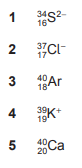6. Which of the following atoms and ions contain(s) 20 neutrons and 18 electrons?
0
1

## Explanation

The number of electrons is equal to the atomic number of the element plus the magnitude of the charge if it is a negative ion or minus the charge if it is a positive ion. The number of electrons for each option are: 1)=16+2=18, 2)=17+1=18, 3)=18, 4)=19-1=18, 5)=20, so eliminate 5). The number of neutrons is given by the mass number minus the proton number: 1)=34-16=8, 2)=37-17=20, 3)=40-18=22, 4)39-19=20, so eliminate 1) and 3). Therefore only 2) and 4) have both 20 neutrons and 18 electrons.

Post CommentAnshika singh Medicmind Tutor

Sat, 28 Aug 2021 15:48:07

Explanation aren't present

A puddle is left on a road after a rain shower. The water in the puddle slowly disappears by evaporation.

Three statements about the effect of changing different conditions on this process are given below.

1 The rate of evaporation is greater at higher temperatures.

2 The rate of evaporation is greater when the air above the puddle is still.

3 The rate of evaporation is greater when the puddle has a larger surface area.

(Assume for each statement that all other conditions remain the same.)

7. Which of the statements is/are correct?
1
1

## Explanation

The first statement is correct because the water molecules have more kinetic energy at higher temperatures, causing them to move faster and further apart to convert to gaseous state. 2 is incorrect because a greater airflow above the puddle would give the water molecules more energy and assist in their evaporation. 3 is correct because the water molecules have a larger area from which they can evaporate per unit time. So statements 1 and 3 only are correct.

Post Comment

In a group of 20 patients at a medical centre, 5 suffered from migraines.

Two patients from the group of 20 were picked at random for a survey on the use of painkillers.

8. What is the probability that both of the patients picked suffered from migraines?
0
0

## Explanation

The probability that the first patient picked suffered from migraines is 5/20. If the first person chosen suffered from migraines, there would be one less person remaining who suffered from migraines and one less person in the group overall, so the probability that the second person picked suffered from migraines would be 4/19. Therefore the probability that both people picked suffered from migraines would be: 5/20 x 4/19= 20/380= 1/19.

Post Comment

The diagram shows four experiments used to investigate movement of substances across dialysis tubing. This tubing is a partially permeable membrane which allows both glucose and water to pass through it.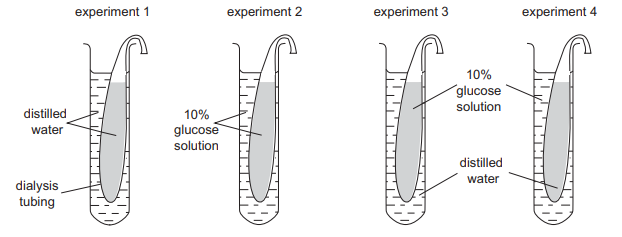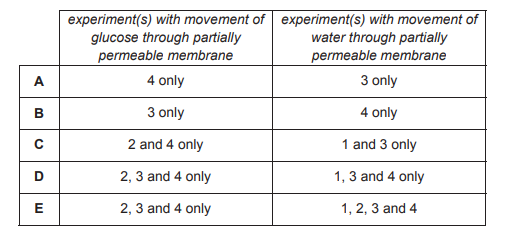9. Which row in the table shows the experiment(s) where there will be movement of glucose through the partially permeable membrane and the experiment(s) where there will be movement of water through the partially permeable membrane?
0
0

## Explanation

In experiments 1 and 2, the same liquid is present on either side of the partially permeable membrane, so there would be no net movement or glucose or water. However, the question is asking about movement not net movement. If a certain type of molecule is present in one/both of the solutions in an experiment, there will be movement regardless of if a concentration gradient exists across the membrane. In experiment 1, there is no glucose present, so there would be no movement of glucose across the membrane. However glucose is present in the remaining experiments, and water in present in all 4 experiments, so the answer is E.

Post Comment

A piece of magnesium ribbon is dropped into a beaker of dilute aqueous hydrochloric acid at room temperature. After a while the production of bubbles of gas slows down.

1 The particles have less energy.

2 The concentration of hydrochloric acid decreases.

3 The activation energy for the reaction increases.

10. Which of the following statements can correctly explain this observation?
0
0

## Explanation

2 is correct because as the reaction progresses, more HCl is used up, so its concentration would decrease. 3 is incorrect as only the presence of a catalyst can alter the activation energy of a reaction. Therefore only the second statement can explain the observation.

Post Comment

Two resistors R1 and R2 are connected in series with a cell.

Resistor R1 has twice the resistance of resistor R2.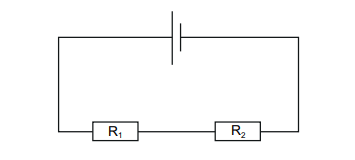1 The voltage across each resistor is the same.

2 The voltage across R1 is twice that across R2.

3 The voltage across R2 is twice that across R1.

4 The current is the same in both resistors.

5 The current in R1 is twice the current in R2.

6 The current in R2 is twice the current in R1.

11. Which pair of statements is correct?
0
0

## Explanation

For a series circuit, the total voltage is determined by the sum of the voltages of each component within the circuit. As the two resistors have different resistances, and because V=IR, they will also have different voltages, so statement 1 is incorrect. According to V=IR, if the resistance doubles whilst the current remains constant, the voltage will also double, so 2 is correct. 3 is the opposite of 2, so 3 must be incorrect. For a series circuit, the current is the same throughout the circuit, so 4 is correct, and hence 5 and 6 must be incorrect because they contradict statement 4. Therefore only statements 2 and 4 are correct.

Post Comment

In triangle PRS, line QT is parallel to side RS.

RS = 1.5 cm

TS = 1.8 cm

QT = 0.3cm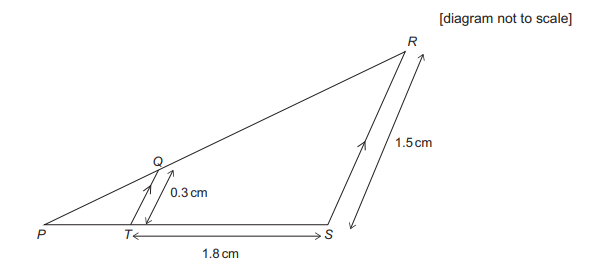12. What is the length of PS?
0
0

## Explanation

To determine the length of PS, we need to find the length of PT and add this to the length of TS given. The triangles PRS and PQT are similar, therefore we can use ratios to determine their side lengths. QT/RS=0.3/1.5=0.2. This will be equal to the ratio of PT/PS. Using algebra: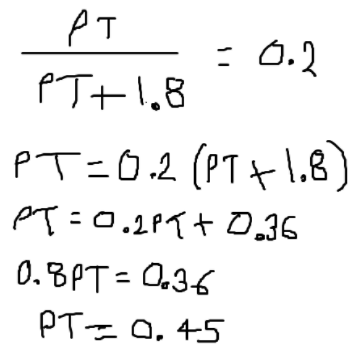Therefore PS=0.45+1.8=2.25cm.

Post Comment

Dolly the sheep was born in 1996. She was unusual because she had no biological father.

Sheep have a diploid number of 54 chromosomes in their body cells.

The diagram shows the process of how she was produced.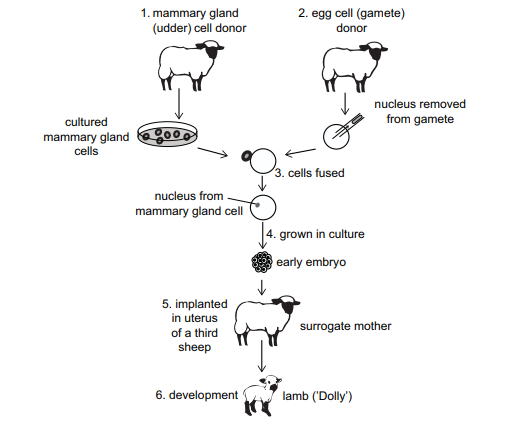1 The gamete cell nucleus contained 27 chromosomes.

2 The cells produced in step 4 had the same properties as stem cells.

3 None of the cells involved in the process were produced by meiosis.

0
0

## Explanation

Gametes contain the haploid number of chromosomes, and since the diploid cell contained 54 chromosomes, the gamete would contain 27, so statement 1 is correct. 2 is correct because the embryo is not yet formed at step 4, so the cells have not yet differentiated. Gametes are produced by meiosis so 3 is incorrect. Therefore only statements 1 and 2 are correct.

Post Comment

Disproportionation is the simultaneous oxidation and reduction of the same species in a reaction.

In which of the following chemical equations does disproportionation occur?

1      Fe + CuCl₂ → FeCl₂ + Cu
2      Cu₂O → Cu + CuO
3      Cl₂ + H₂O → HCl + HClO
4      BaCl₂ + Na₂SO₄ → BaSO₄ + 2NaCl
5      Hg2Cl₂ → Hg + HgCl₂

14. In which of the following chemical equations does disproportionation occur?
0
0

## Explanation

In reaction 1, iron is being oxidised (loses electrons) and copper is being reduced (gains electrons), so this is not a disproportionation reaction. In 2, copper is both oxidised (to 2+) and reduced (to 0), so this is a disproportionation reaction. In 3, chlorine is both oxidised (to +1) and reduced (to -1). In 4, the oxidation states of all the elements are unchanged. In 5, Hg+ is oxidised (to 2+) and reduced (to 0), so this is a disproportionation reaction. Therefore, the reactions 2, 3 and 5 only are disproportionation reactions.

Post Comment

Uranium-238 is a naturally-occurring alpha emitter. It can be used in the manufacture of the isotope plutonium-239, during which it is bombarded by neutrons.

The process of converting a nucleus of uranium-238 to a nucleus of plutonium-239 is a three-stage sequence of nuclear reactions.

(Atomic numbers: uranium = 92; plutonium = 94)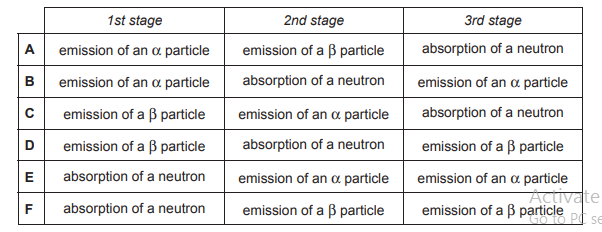15. What is this sequence?
0
0

## Explanation

When converting uranium-238 to plutonium-239, the change in mass number is 1+ and the atomic number changes by 2+. We know that the 3 stages cannot involve emission of an alpha particle because this would cause a reduction in mass number of 4 and a reduction in atomic number of 2, after which the element cannot be altered by the other two stages in a way which would give the desired mass and atomic numbers. Therefore we can eliminate A,B,C and E. This leaves D and F, which both have the same events but in different orders. F is the correct answer because the absorption of a neutron in stage 1 would result in the emission of the beta particles.

Post Comment

The acceleration due to gravity at the surface of a planet is given by g = GM/R² , where M and R are the mass and radius of the planet respectively, and G is the gravitational constant.

It is given that

g = 10N/ kg

G = 7 × 10 ̵¹¹ Nm² / kg²

R = 6 × 10⁶ m

16. What is M, correct to one significant figure?
0
0

## Explanation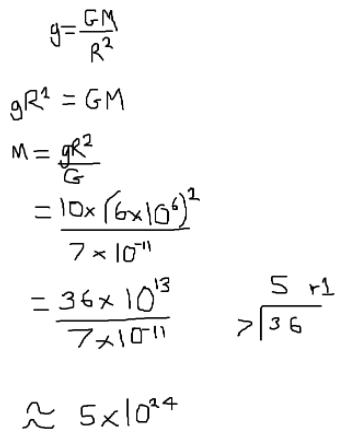Post Commentsammy Medicmind Tutor

Wed, 27 Oct 2021 20:23:16

this is formatted incorrectly so you cant see that one of the powers is negative

The diagram shows an external view of the human heart.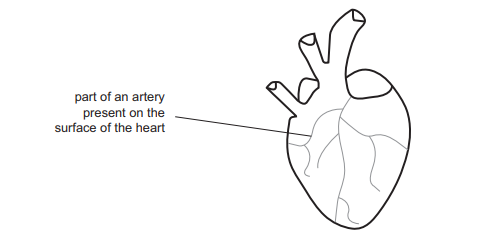1 It is the site of diffusion of glucose and oxygen into the muscle cells of the heart.

2 It transports blood at high pressure.

3 It contains muscle cells.

17. Which of the following statements about the artery shown on the diagram is/are correct?
0
0

## Explanation

The artery shown is a coronary artery. Statement 1 is incorrect because artery walls are impermeable so glucose and oxygen would not diffuse through them. 2 is correct because arterial blood pressure is generally high, as the blood has come directly from the heart and not yet been slowed down by passing through tissues. 3 is also correct because there is smooth muscle present in arterial walls which contract to cause vasoconstriction. Therefore, statements 2 and 3 only are correct.

Post Comment

Propanoic acid is a monoprotic acid.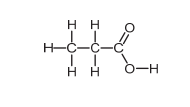Magnesium is a Group 2 metal.

18. Which of the following chemical equations is correct for the reaction between magnesium carbonate and propanoic acid?
0
0

## Explanation

Propanoic acid is a monoprotic acid, which means is loses only 1 proton (H+) per molecule when it dissociates, forming an ion with a 1- charge. However, because Mg is a group 2 metal, it will lose 2 electrons when it reacts to form a 2+ ion. Therefore, in order for the number of electrons and hence charge to remain balanced, 2 moles of propanoic acid must react with every 1 mole of magnesium. Therefore the answer must be A, C or D. Because only C is balanced correctly, C is the answer.

Post Comment

A swimming pool is 10 m wide. A loud sound is made in the water 2.0 m from one wall and the reflected sounds are detected with a microphone placed next to the sound source. The reflection from the wall 8.0 m away arrives 0.010s after the reflection from the wall 2.0 m away.

19. What is the speed of sound in water?
0
0

## Explanation

The sound made travels 2m to the closest wall and then 2m back to the detector placed at the sound source, but also travels 8m in the opposite direction to the further wall and then travels 8m back to the detector. Therefore, the reflection from the wall 2m away travels 4m, and the reflection from 8m away travels 16m. Because the second sound detected travelled 16/4=4 times the distance of the first sound, it would have taken 4 times the amount of time taken for the first sound to be detected (as the speed of sound in water is constant). We can form an equation where x is time taken for the first sound to be detected:

4x-x=0.010

3x=0.010

x=0.010/3

Speed=distance/time, and the first sound detected travelled 4m so:

4/(0.010/3)

=12/0.010

=12×10²

=1200 m/s

Post CommentLeona Medicmind Tutor

Tue, 02 Nov 2021 22:49:38

in the first sentence, it should be "wave", not "made"

Express    1/2x    +    1/x-1    –    1/x    as a single algebraic fraction.

20.
1
0

## Explanation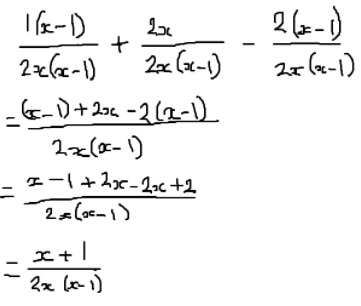Post Comment

The family tree shows the inheritance of freckles.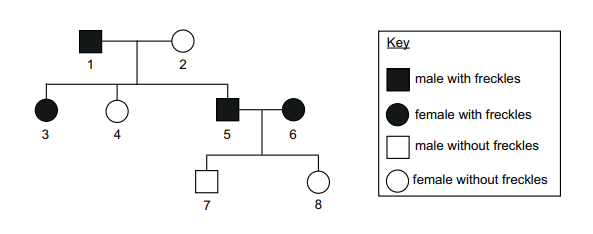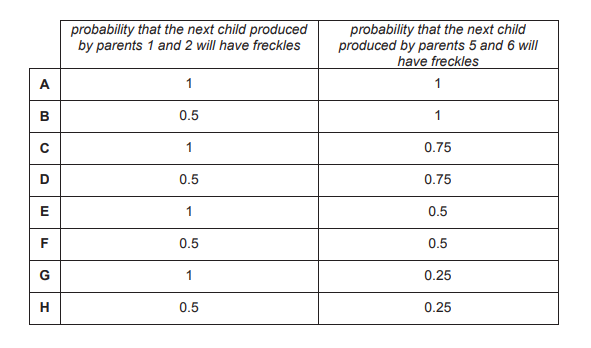21. Which row in the table shows the probability that the next child produced by parents 1 and 2, and the probability that the next child produced by parents 5 and 6 will have freckles?
0
0

## Explanation

The allele causing the presence of freckles must be dominant (F) because 5 and 6 both have freckles but neither of their children do, so 5 and 6 must also each have the recessive allele for no freckles (f), which they passed onto their offspring. The genotype of 2 is therefore ff. The genotype of 1 must be Ff, because 1 and 2 have children both with and without freckles. In the cross Ffxff, the 2 of the offspring would have freckles and 2 would not, so the probability of a child produced having freckles is 0.5. The genotype for both 5 and 6 is Ff, and a heterozygous cross produces offspring with phenotypes in the ratio of dominant:recessive=3:1, so the probability that one of their offspring has freckles is 0.75. Therefore the answer is D.

Post Comment

Hydrated copper(II) sulfate has the formula CuSO₄·5H₂O.

(Ar values: H = 1.0; O = 16; S = 32; Cu = 64)

22. 100 cm³ of a solution contained 10 g of hydrated copper(II) sulfate. What is the concentration, in mol / dm³ , of this solution?
0
0

## Explanation

64+32+(16×4)+5((1×2)+16)=64+32+64+90=250

Mass/Mr=moles: 10/250=0.04mol

100cm³=0.1dm³

Concentration=moles/volume: 0.04/0.1=0.4moldm³

Post Comment

A book rests on a table, which in turn rests on the floor. The floor exerts a force P on the table.

Force P and one other force constitute a Newton’s Third Law interaction pair of forces.

23. What is the other force?
0
0

## Explanation

Newton’s third law is: whenever two objects interact, they exert equal and opposite forces on each other. The force acting opposite to force P is the force that the table exerts on the floor, which is answer F.

Post Comment

The diagram shows a shape made from a quarter circle of radius 6cm and a right-angled triangle with a hypotenuse of length 9 cm.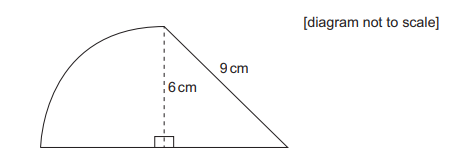24. Which of the following expressions gives the area, in square centimetres, of the shape?
0
0

## Explanation

The area of the triangle is given by 1/2 x base x height. Use pythagoras to work out the length of the base: 9² – 6² = 81 – 36 = 45, so base=√45=3√5cm. So the area of the triangle is: 0.5 x 3√5 x 6 = 9√5. The area of the quarter circle is given by 1/4 x : 0.25 x π x 6 x 6 = 9. So the total area is + 9√5.

Post Comment

The picture shows a Siamese cat. This type of cat has a gene which is sensitive to temperature. When this gene is inactive in the cells, the coat colour is pale. When the gene is active, it produces an enzyme which causes the coat colour to be darker. If this cat had grown up in a warmer environment its ears, front of face, paws and tail would be paler than those shown in the picture below.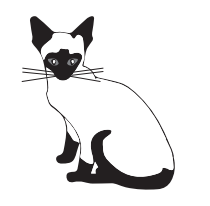A student wrote the following statements using this information.

1 The enzyme is denatured at the internal body temperature of the cat so the coat colour is pale.

2 The temperature of the ears, front of face, paws and tail was cooler than the body temperature so they are darker.

3 The coat colour in Siamese cats depends on both genes and the environment.

25. Which of these statements is/are correct?
0
0

## Explanation

Enzyme activity causes a darker coat. If this cat had grown up in a warmer environment its ears, front of face, paws and tail would be paler, which means the enzyme is inactivated at higher temperatures. Statement 1 is incorrect because the coat of the cat is superficial, so the enzyme would not be working at internal body temperature. 2 is correct as we know that a darker coat is caused by increased enzyme activity. Statement 3 is correct because the enzyme is coded for by a gene but its activity is affected by temperature. Therefore statements 2 and 3 only are correct.

Post Comment

What is the volume of hydrogen gas formed (measured at room temperature and pressure) when 0.23 g of pure sodium reacts completely with an excess of water?

(Ar values: H = 1.0; Na = 23.

Assume that the molar volume of gas at room temperature and pressure is 24 dm³ .)

26.
1
0

## Explanation

Na+H2O→ NaOH+1/2H2

Moles=mass/Mr: 0.23/23=0.01mol of Na

0.01/2=0.005mol of H2

0.005×24=0.12dm³

Post Comment

A graph of kinetic energy, in joules (y -axis) against the square of the speed in (m / s)² (x -axis) is plotted for an object of mass 2.5 kg travelling along the surface of the Earth. The result is a straight line.

27. What is the numerical value of the gradient of this line?
0
0

## Explanation

Kinetic energy=1/2mv².

KE=0.5×2.5v²

KE=1.25v²

The kinetic energy is directly proportional to the square of the velocity (or speed with direction) according to the equation. As KE and v are the variables, 1.25 must be the gradient of the line. Comparing the equation  to y=mx²+c, KE=y, v=x and 1.25=m

Post Comment

## Instructions

Below is a summary of your answers. You can review your questions in three (3) different ways.

The buttons in the lower right-hand corner correspond to these choices:

2. Review questions that are incomplete.
3. Review questions that are flagged for review. (Click the 'flag' icon to change the flag for review status.)

You may also click on a question number to link directly to its location in the exam.

## Instructions

This review section allows you to view the answers you made and see whether they were correct or not. Each question accessed from this screen has an 'Explain Answer' button in the top left hand side. By clicking on this you will obtain an explanation as to the correct answer.

At the bottom of this screen you can choose to 'Review All' answers, 'Review Incorrect' answers or 'Review Flagged' answers. Alternatively you can go to specific questions by opening up any of the sub-tests below.

x

## When should we call you?

It would be great to have a 15m chat to discuss a personalised plan and answer any questions

## What time works best for you? (UK Time)

Pick a time-slot that works best for you ?

Submit

Submit